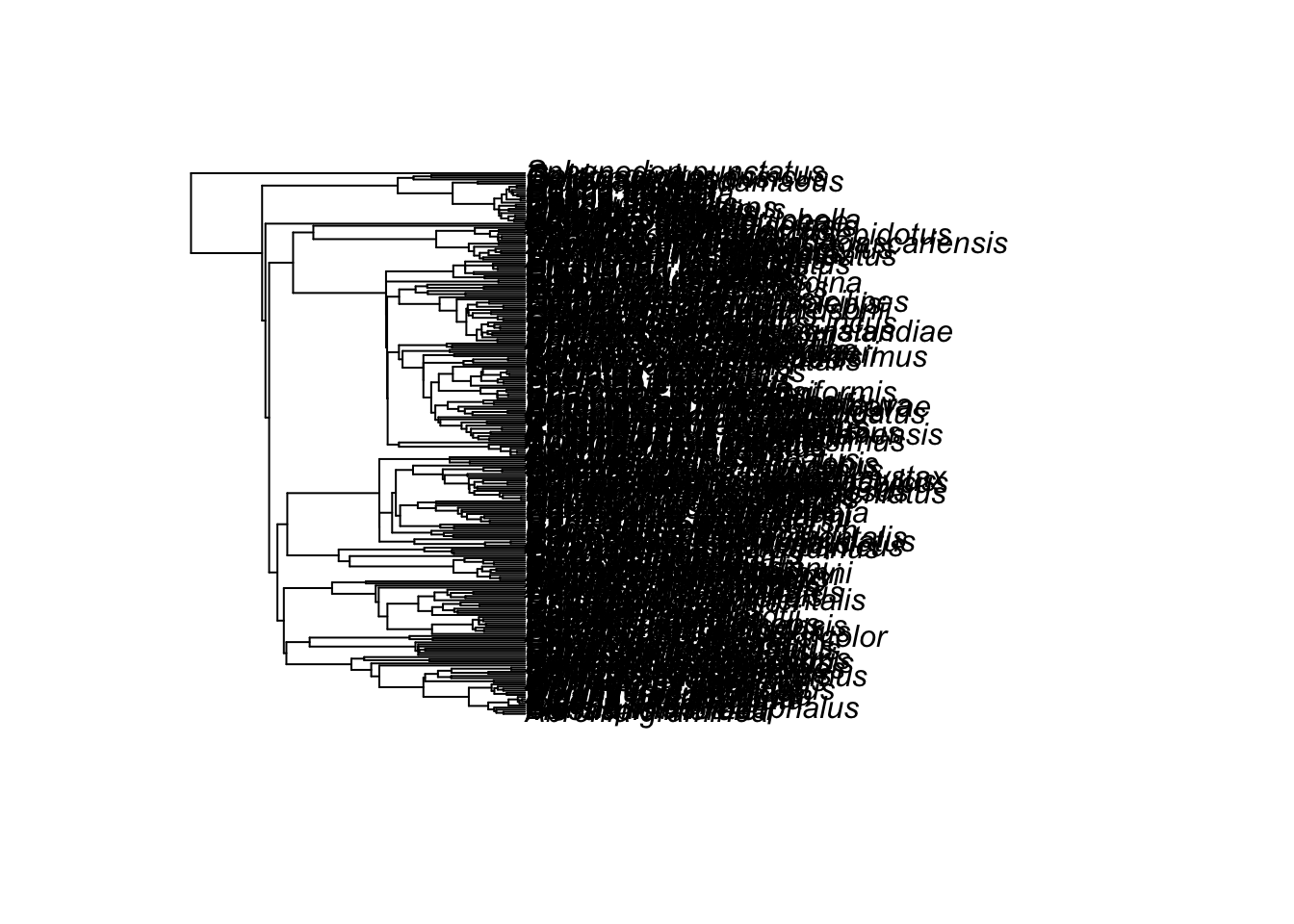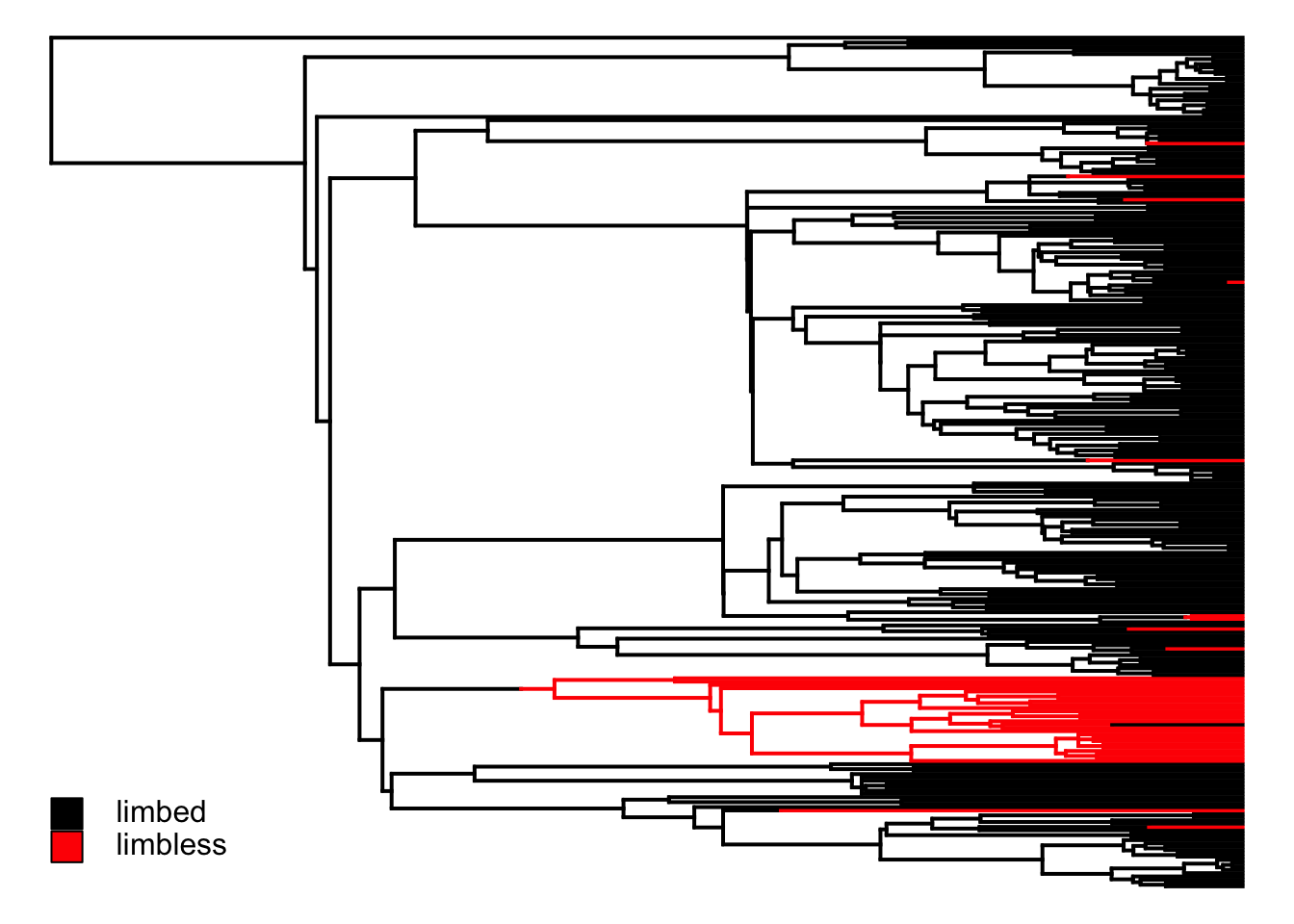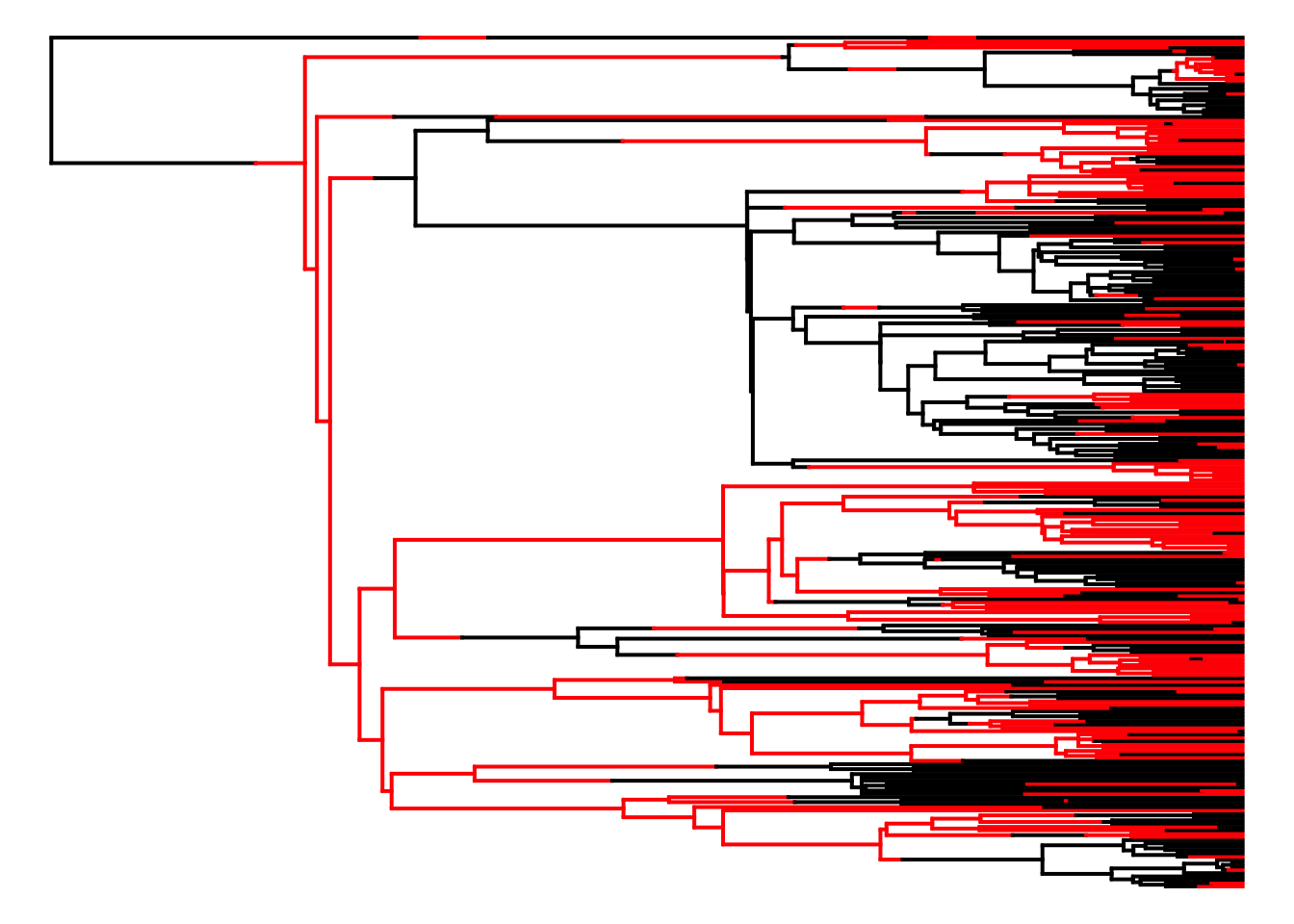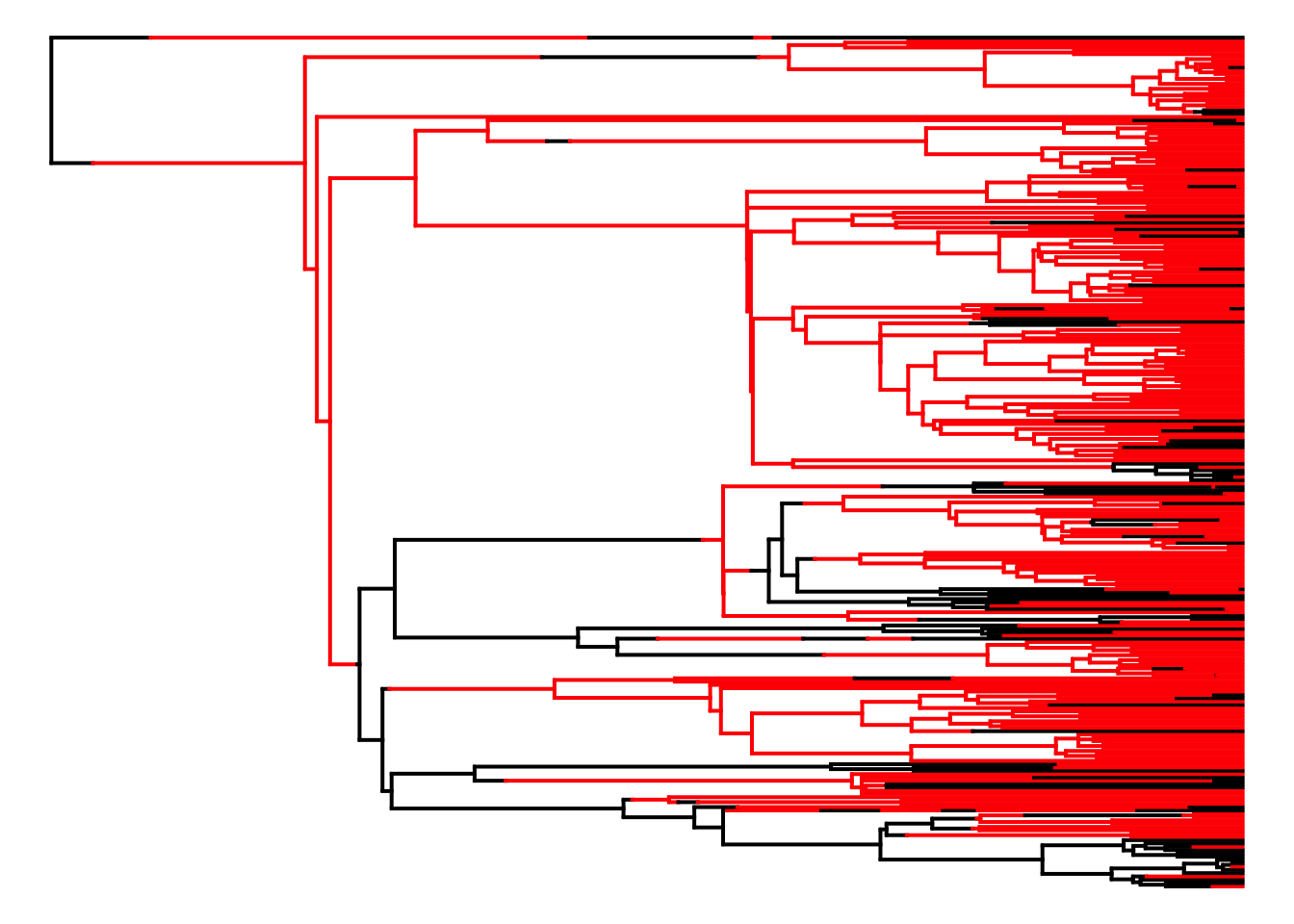# 7.6: R Markdown to Recreate Analyses

R markdown to recreate analyses

## Reading in the data files

First we read in the data files.

sqTree<-read.tree(text=getURL("https://raw.githubusercontent.com/lukejharmon/pcm/master/datafiles/squamate.phy"))
plot(sqTree)sqData<-read.csv(text=getURL("https://raw.githubusercontent.com/lukejharmon/pcm/master/datafiles/brandley_table.csv"))

## Simulate binary character on tree

This code generates plots like Figure 7.4

qMatrix<-cbind(c(-1, 1), c(1, -1))*0.001
sh_slow<-sim.history(sqTree, qMatrix, anc="1")
## Done simulation(s).
plotSimmap(sh_slow, pts=F, ftype="off")
## no colors provided. using the following legend:
##       1       2
## "black"   "red"
add.simmap.legend(leg=c("limbed", "limbless"), colors=c("black", "red"), x=0.024, y =23, prompt=F)qMatrix<-cbind(c(-1, 1), c(1, -1))*0.01
sh_fast<-sim.history(sqTree, qMatrix, anc="1")
## Done simulation(s).
plotSimmap(sh_fast, pts=F, ftype="off")
## no colors provided. using the following legend:
##       1       2
## "black"   "red"qMatrix<-cbind(c(-0.02, 0.02), c(0.005, -0.005))
sh_asy<-sim.history(sqTree, qMatrix, anc="1")
## Note - the rate of substitution from i->j should be given by Q[j,i].
## Done simulation(s).
plotSimmap(sh_asy, pts=F, ftype="off")
## no colors provided. using the following legend:
##       1       2
## "black"   "red"## Find the limbless species

Brandley et al.’s data has limb measurements. We will get our discrete character by counting species with zero-length fore- and hind limbs as limbless. This is different from the original analysis in Brandley et al., which counts things like spurs as “limbs” - and so our results might differ from theirs a bit.

limbless<-as.numeric(sqData[,"FLL"]==0 & sqData[,"HLL"]==0)
sum(limbless)
##  51
# get names that match
nn<-sqData[,1]
nn2<-sub(" ", "_", nn)
names(limbless)<-nn2

### Fit Mk model

We can fit a symmetric Mk model to these data using both likelihood and MCMC

# likelihood
td<-treedata(sqTree, limbless)
## Warning in treedata(sqTree, limbless): The following tips were not found in 'phy' and were dropped from 'data':
##  Gonatodes_albogularis
##  Lepidophyma_flavimaculatum
##  Trachyboa_boulengeri
dModel<-fitDiscrete(td$phy, td$data)

# MCMC
mk_diversitree<-make.mk2(force.ultrametric(td$phy), td$data[,1])
simplemk<-constrain(mk_diversitree, q01~q10)
er_bayes<-mcmc(simplemk, x.init=0.1, nsteps=10000, w=0.01)
## 1: {0.0189} -> -141.93605
## 2: {0.0162} -> -135.45732